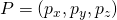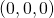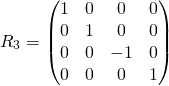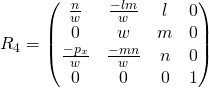# magnification

a) For telecentric lenses this is  the ratio of image size to object size
b) For entocentric lenses this is  the ratio of image size to object size at a given distance.

Example (telecentric lens)
If you want to map an obbject of 10mm diameter to s Sensor with 1/3″ (= 6mm diagonal!), you need a magnification of 6/10 = 0.6x
The lower the magnification, the larger the visible object section. Say, if you can’t get a lens with the desired magnification, you can choose a lens with a slightly smaller magnification, eg 0.55x instead of 0.6x in the above example.
Example (entocentric lens)
With a 1/2 “Sensor (8mm diagonal), a distance of 500mm and an object cutout of 16mm diagonal,   the magnification is  8/16 = 0.5.
Doubling the distance (to 1000mm) allows the lens to see about twice as much (32mm ). As a result, a magnification of 8/32 = 0:25 results.
In particular, the magnification at infinity is zero!
Since for entocentric lenses the FOV changes with the working distance, the magnification changes too!
Each entocentric lens achieves each magnification (if it can be used beyond the MOD)! .. We just have to choose the right distance between the object and the camera.
So there’s the naive hope that just one entocentric lens could be enough for all applications then …
The Problem is however, that (for entocentric lenses) with the distance to the object, also the perspective changes. Telecentric lenses keep the perspective!Typical high magnifications in image processing end at 10x.
Typical high magnifications in microscope imaging end at 100x, where magnifications above 40x usually need immersion, say, the lens is used in oil!.
When you read about higher magnifications like 200x and higher, there’s an excellent chance that the size of the monitor is also included!

# main optical axis

The Symmetry axis of a lens or a lens system.

# Reflection at a plane in 3D

A flat mirror in 3D is descibed by the direction cosinesof a surface normal and a point P on it’s surface.

We construct the image A’ of a point A by these steps

• translate the origin of the coordinate System to the point P
• rotate the coordingate system so, that the z-axis of the coordinate system coincides with the surface normal in P)
• mirror point A in this new coordinate system
• unrotate the coordinate system to it’s old rotation
• untranslate the origin to it’s old position

## Translation of the coordinate system in 3D so that the Origin is in P.whereare the cartesian coordinates of point P=()

Proof thatis indeed mapped to:## Rotation in 3D around the Origin (now in P) so that the z-axis coincides with the surface normalwithProof, that each pointis mapped to## Reflection at the z-axis in 3D## De-Rotation in 3Dwith## Translation in 3D back to original Position# Reflection at a plane in 3D

*** QuickLaTeX cannot compile formula:
R = R_5 R_4 R_3 R_2 R_1
<span class="ql-right-eqno">   </span><span class="ql-left-eqno">   </span><img src="https://www.optowiki.info/wp-content/ql-cache/quicklatex.com-fd64c24ef2bdf7310c7a75a3f6537980_l3.png" height="88" width="419" class="ql-img-displayed-equation quicklatex-auto-format" alt="$= \begin{pmatrix} 1-2l^2 & -2lm & -2ln & 2l(lp_x + mg + nh) \\ -2lm & 1-2m^2 & -2mn & 2m(lpx + mg + nh) \\ -2ln & -2 m n & 1-2n^2 & 2n(lpx + mg + nh) \\ 0 & 0 & 0 & 1 \end{pmatrix}$" title="Rendered by QuickLaTeX.com"/>
<span class="ql-right-eqno">   </span><span class="ql-left-eqno">   </span><img src="https://www.optowiki.info/wp-content/ql-cache/quicklatex.com-b17224e260f969ba19e366728afd8719_l3.png" height="88" width="301" class="ql-img-displayed-equation quicklatex-auto-format" alt="$= \begin{pmatrix} 1-2l^2 & -2lm & -2ln & 2lp \\ -2lm & 1-2m^2 & -2mn & 2mp \\ -2ln & -2 m n & 1-2n^2 & 2np \\ 0 & 0 & 0 & 1 \end{pmatrix}$" title="Rendered by QuickLaTeX.com"/>
Where

*** Error message:
Missing $inserted. leading text: R = R_  p = (lp_x + mp_y + np_z) =$ length from the origin to point P

# mechanical vignetting

Mechanical obstacles in the path of light cause a drop in brightness (“artificial vignetting”).

A drop in brightness can also happen unintentionally (and thus may be a design mistake). You can correct it usually by stopping down the lens (smaller the iris opening)  by for example  2-3 f-stops)

Looking from the front to a lens has a certain resemblance to axially look into a cylindrical tube.
At the other end of the tube can be seen a circle, namely, the end of the tube.The small green or red circle above suggests doing a stopped-down lens (= lens with a large aperture number).

Tilting the tube (lens) slightly off-center, for some degree a complete circle remains visible ( marked green in the drawing above).
We see, that for small circles (= large f-numbers) the tube (=lens) can be tilted more before the full circle touches the edge of the cylinder.

When we tilt the tube (=lens) (lens) more,  the complete circle is no longer visible,, marked in red.

That fact that the complete circle is visible is a sign that in this direction the entire light can pass through the tube.
The full circle turns into a “biangle” (= intersection of two circles).

This is a sign that in this direction not all of the light can pass the cylinder (=the lens). Vignetting occurs.
The percentage of light passing is

100 * (area of the biangle / area of ​the ​circle

When we connect the image points of a sensor and the corresponding object points, This line has an angular displacement from the optical axis. The angle corresponds to the deflection of the cylinder above.

Mechanical vignetting occurs symmetrically and therefore first occurs in the corners of the sensor.

When we tilt the tube (=lens) too much, no light passes.

The area on the sensor receiving light  is round (due to circular symmetry reasons) and is called  “image circle”.
Black areas are usually to be avoided in the image, say,  the image circle got to be larger than the sensor diagonal.

If the image circle is slightly large than 6mm, it’s called a lens 1/3″ lens.
At over 8mm it’s a so called  1/2 ” lens, etc.

see MOD

# MOD

= Minimum object distance
Closest distance for which the lens works optimal and especially can be focused.

This does not mean that a lens at shorter working distances no longer “works”. … just: please do not expect the “perfect” picture quality.

Focus on shorter working distances can be achieved by increasing the distance between sensor and lens (= screw type design of the lens and possible use of distance rings)

When working at shorter distances than MOD generally expect:

• The light sensitivity changes.   I.e. Images are generally darker because less light reaches the sensor.
• Depending on the very lens design, it may happen that mechanical vignetting occurs, because  unintentionally   other mechanical components in the objective take on the role of the diaphragm.
• Field curvature may increase. You can tell from focusing that is only locally, say, focus only on the edge, or just in the middle or only on a ring around the center.

# monitor magnification

describes, how much larger an object is displayed on a monitor than it is in real life.May the object width be 1.6mm and the sensor width be 4.8mm.
May the sensor diagonal be 6mm and the monitor diagonal = 50″ = 128cm = 1280mm.

ThenandBecausewe get :